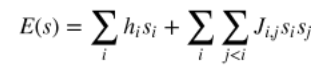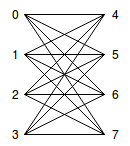# How does my problem get processed in a quantum computer?

I have looked at some of the Leap demos and have perused over the open source code. Just to keep things organized in my head, could someone please give me a high level overview on what is happening? How does my problem get processed on the quantum computer? What are the general steps to programming my problem onto the QPU?

1 comment
• General concept: When we measure qubits, they will tend to orient themselves so that the overall system will be at its lowest energy state. This is something that happens naturally. So the main idea here is to set up the system so that it corresponds to the problem that we are interested in, so that the measured state of the qubits in this system will correspond to the solution to our problem. In other words, convert our problem into an minimization problem, that way, the state of the qubits will be the solution to this problem.

General steps that occur when programming on a quantum processor:

1. Transform your problem into an Ising or QUBO (the type of optimization problem that the quantum processor can solve)
2. Transformed problem gets put into the quantum processor
3. Quantum processor gets sampled (aka qubits get measured; these measurements are potential solutions to the problem)
4. Samples (potential solutions) get returned back to the user

Note on Step 1: If you can express your problem as a constraint satisfaction problem (CSP), there are functions in the Ocean toolkit (specifically, `dwavebinarycsp`) that will transform your CSP into a Ising or QUBO problem. That is, the Ocean toolkit will take your CSP and return the necessary coefficients (h and J variables) to Ising/QUBO equation (see equation below). This equation will later get fed into the quantum processor, which would provide the s variables that correspond to low energies.Another note on Step 1: Ising and QUBO problems fall under the umbrella of a more general term, Binary Quadratic Models (BQM). The term BQM will come up a lot in the code, but it is just a general term referring to Ising and QUBO problems.

Note on Step 2: When the problem gets put into the quantum processor, the problem is said to be embedded into the processor. Embedding is particularly interesting, so I will briefly describe it here as well.

• Ising and QUBO problems can be depicted as a graph. Naively, we would expect the nodes of this graph to be represented by qubits, while the edges to be represented by a dependency formed between said qubits.
• However, the hardware in the quantum processor is constructed in a particular way; there are limitations to the number of couplings (edges) that could be formed per qubit as well as which qubits can form a coupling together. For example, consider the eight qubits (labelled 0 to 7) in the figure below. Each qubit has a limited number of edges, and these edges are only formed with particular qubits (ex. Qubit 0 can be coupled with qubits 4 to 7, but not with qubit 1).• Since the original BQM (i.e. Ising or QUBO problem) does not necessarily conform to the way the QPU hardware is connected, the embedding process is a way of transforming the original BQM so that it fits onto the quantum processor. For example, in the figure above, if the original graph required qubit 0 to be coupled with qubit 1, then the embedding process may have qubit 4 act as a 'clone' of qubit 1 (i.e. form a strong coupling between qubits 1 and 4). That way, the interactions that qubit 0 has with qubit 4 (the clone), would be as if qubit 0 was directly interacting with qubit 1.
• The user does not need to deal with this embedding process directly. It is something that gets taken care of when `EmbeddingComposite(..)` from `dwave.system.composites` gets called.

Additional information can be found on https://docs.ocean.dwavesys.com/en/latest/overview/solving_problems.html